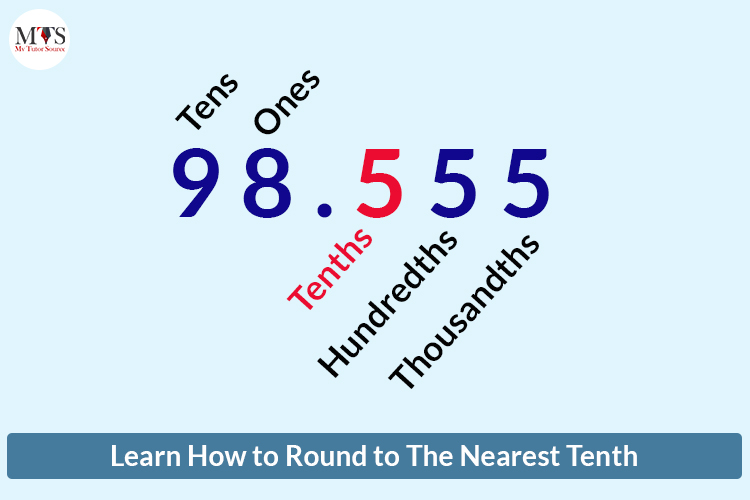# Learn How to Round to The Nearest Tenth

• Published On:Life gets easy once you learn decimal rounding methods, and using them every day will become a part of your routine. Whether you are estimating your shopping bills, balancing accounts or rounding numbers saves time. In this blog post, you will learn how to round a decimal to the nearest tenth.

## Rounding Number

Let’s start with what rounding off numbers means. It is a mathematical technique to adjust the decimal place numbers to a particular degree of accuracy to make them easier to use, calculate and understand. They are not the exact correct answer but are still very close to the right or original value.

Decimal numbers or places come right after the decimal point. Let’s learn what ones, tenths, or hundredths digit represents with examples.

Ones digit: it represents the whole numbers and is generally used in daily life to count things, like the number of tomatoes (2.0 tomatoes = two tomatoes)

Tenths digit: it represents one-tenth of something or an item if you cut a cake into 10 equal pieces to distribute (0.2 of a cake = two pieces of a 10-piece cake)

Hundredths digit: it represents one-hundredth of something, like currency such as a cent is worth of one one-hundredth of a dollar (1 cent=\$0.01)

## Round To The Nearest Tenth

While rounding numbers to the nearest digit, look for the numbers right next to its decimal point. For rounding to the nearest tenths, look which digit is right to the tenth place. If it is between 5 and 9, then round up, or if it is 4 or lower, the digit will not change.

### For Example:

• 6.57 can round to 6.6 because the hundredth-place digit is 7, which lies between 5 and 9.
• 9.81 would not round up or down because the hundredth place 1 is less than 5.

## Round To The Nearest Hundredth

The same technique is for rounding to the nearest hundredths, but you have to look for the thousandths place digit this time.

### For Example:

• 29.446 can round to 29.47 because the thousandth place is 6 and lies between 5 to 9.
• 98.555 would also round to 98.56 because the thousandth place is 5.

## Round Across the Decimal Place

Rounding to the units or ones place means rounding across the decimal place or nearest whole number. Look to the right next to the decimal point, called the tenth place, to round off the ones or unit digits.

### For Example:

• 9.9 rounds to 10 because 9 lies between 5 and 9.
• 3.2 rounds to 3 because 2 is less than 5.

### Rules To Remember

• Always look at the right side of the decimal point for rounding off digits.
• For rounding the tens digit, look at the nearest hundredths digit and for rounding the thousandth, look for the nearest ten-thousandths digit.
• If the digit right to the rounding digit is 5 or higher, then the rounding digit will change.
• If the digit right to the rounding digit is 4 or lower, the rounding digit will not change.

Want to practice more examples of Rounding Decimal Numbers? Learn from our expert Math tutors. We give the best tutoring experience.

Take a free trial now and get ready to enhance your knowledge and achieve targeted goals.

### Find Top Tutors in Your AreaAustin has 10+ years of experience in teaching. He has researched on thousands of students-related topics, issues, and concerns. You will often find him writing about the common concerns of students, their nutrition, and what is beneficial for their academics and health both.